GFG App
Open AppBrowser
Continue

There are two Greek words that make up the word polyhedron, the first is ‘poly’ meaning many, and the other is ‘hedron’ meaning surface. Generally, any solid shape with flat faces and straight edges is referred to as a polyhedron. Since the name includes ‘poly’ as a prefix, thus, the name of the shape depends on the number of faces present in the particular shape. For example, A polyhedron with four faces is known as Tetrahedron.

### Introduction to Polyhedral Graph:

A polyhedral graph is a network connecting various concepts or variables. In a polyhedral graph, each vertex has n edges. Polyhedral graphs are undirected graphs whose edges and vertices form convex polyhedrons. Polyhedral graphs, on the other hand, are purely graph-theoretical, connected 3-vertex graphs. Refer to the attached figure for a better understanding.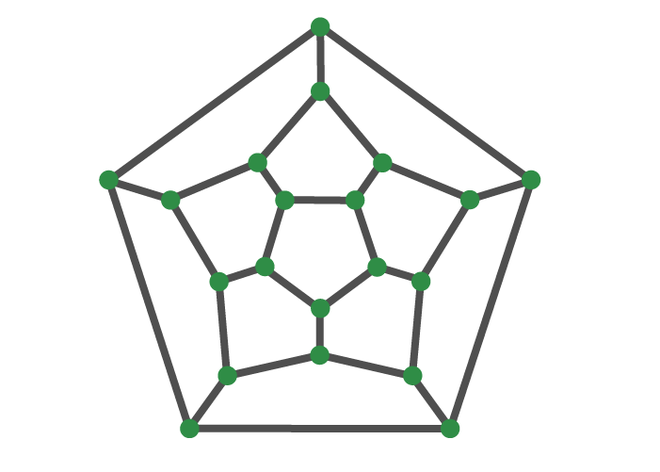Polyhedron Graph

### Characteristics of Polyhedral graph:

A polyhedron comprises the following properties,

• A polyhedron’s faces are the flat surfaces that make up the polyhedron. These are normal polygonal faces.
• The edges are the locations where two flat surfaces meet to produce a line segment.
• The vertex is the point at which the polyhedron’s edges intersect. A vertex is also known as a polyhedron’s corner.
• A polyhedral graph is one created from the vertices and edges of a convex polyhedron.
• Every polyhedron satisfies Euler’s formula or polyhedron formula.

F + V = E + 2,  (here F refers to faces, V refers to vertices and E refers to edges)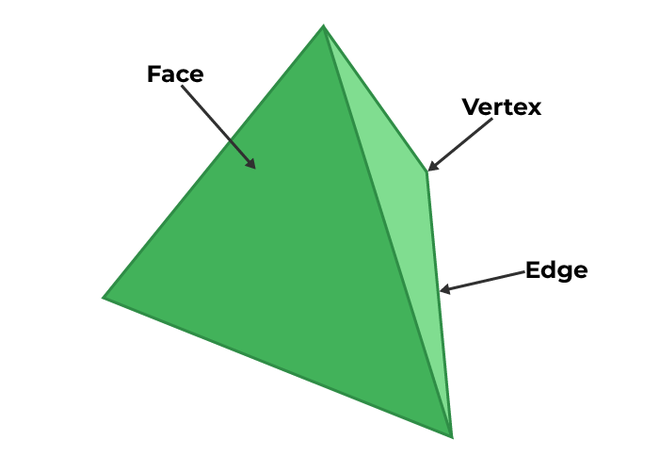Parts of Polyhedron

### Types of Polyhedrons:

The structure of a polyhedron can be further classified based on the elements that form it (Vertices, Faces, and Edges) into the following subcategories.

• Regular Polyhedron
• Irregular Polyhedron

Refer to images for diagrammatical representations.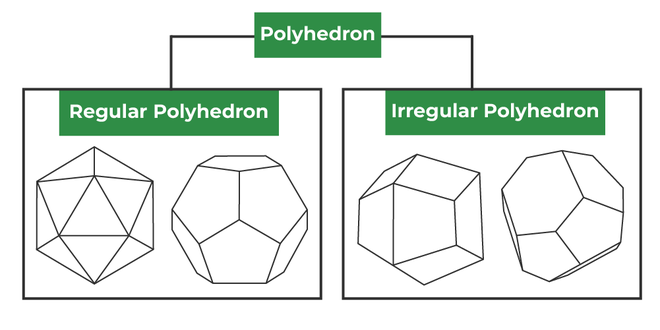Types of Polyhedron

Regular polyhedron: A regular polyhedron is made up of regular polygons that are arranged in a regular pattern. The term “platonic solid” is also used to describe such solids. These polyhedrons are congruent in nature. In other words, we can say the set of polygons that are combined together to form a regular polyhedron is congruent with each other. Refer to the attached figure for a better understanding.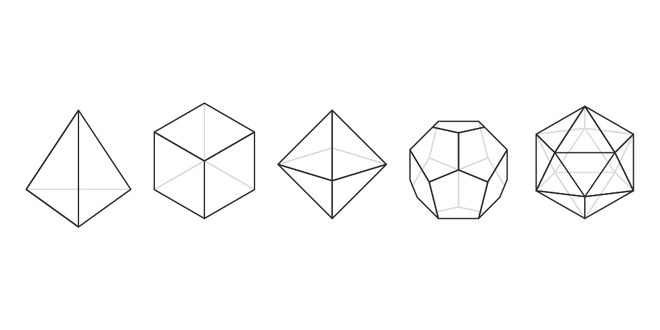Regular Polyhedron

Irregular Polyhedron: An irregular polyhedron is formed by polygons with non-identical shapes, and these polygons make up a polyhedron with an asymmetric shape. There is a difference in size between polyhedrons with irregular faces and those with regular faces. In other words, the combination of different types of polygons together forms an irregular polyhedron. Refer to the attached figure for a better understanding.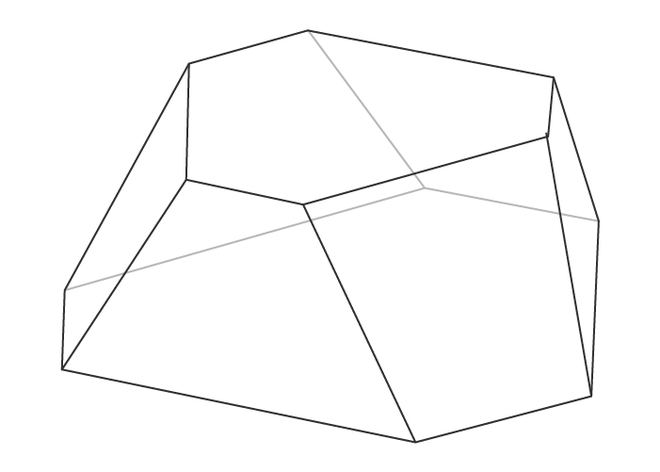Irregular Polyhedron

### Dual polyhedron:

As the significance itself says, a polyhedron originates from another structure in the association. This relationship is formed by the joining of one’s vertices and the other’s faces. Refer to the attached image for a pictorial representation.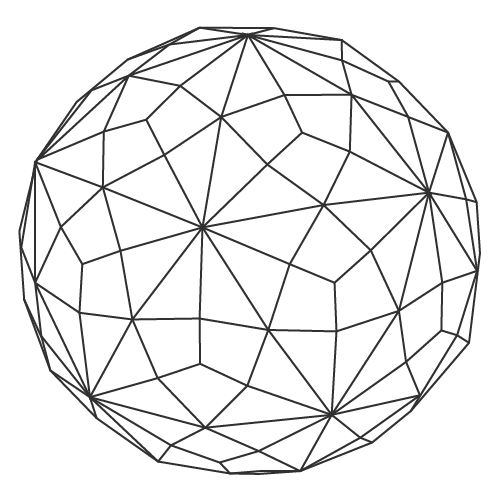Dual Polyhedron

### Example:

There are a lot of examples of polyhedrons available around us in our daily lives. A few of them are as follows:

1. Prism: It is formed by joining three rectangles followed by joining two triangles as the base.
2. Cube: A cube is formed by joining 6 squares together.
3. Pyramid: A tetrahedron is a polyhedron with four sides, also known as a pyramid.
My Personal Notes arrow_drop_up